Numerical integration, quadrature for various domains

## Project description

Your one-stop shop for numerical integration in Python.

More than 1500 numerical integration schemes for line segments, circles, disks, triangles, quadrilaterals, spheres, balls, tetrahedra, hexahedra, wedges, pyramids, n-spheres, n-balls, n-cubes, n-simplices, the 1D half-space with weight functions exp(-r), the 2D space with weight functions exp(-r), the 3D space with weight functions exp(-r), the nD space with weight functions exp(-r), the 1D space with weight functions exp(-r2), the 2D space with weight functions exp(-r2), the 3D space with weight functions exp(-r2), and the nD space with weight functions exp(-r2), for fast integration of real-, complex-, and vector-valued functions.

### Installation

pip install quadpy


See here on how to get a license.

Quadpy provides integration schemes for many different 1D, 2D, even nD domains.

To start off easy: If you'd numerically integrate any function over any given 1D interval, do

import numpy as np

def f(x):
return np.sin(x) - x



This is like scipy with the addition that quadpy handles complex-, vector-, matrix-valued integrands, and "intervals" in spaces of arbitrary dimension.

To integrate over a triangle, do

import numpy as np

def f(x):
return np.sin(x) * np.sin(x)

triangle = np.array([[0.0, 0.0], [1.0, 0.0], [0.7, 0.5]])

# get a "good" scheme of degree 10
val = scheme.integrate(f, triangle)


Most domains have get_good_scheme(degree). If you would like to use a particular scheme, you can pick one from the dictionary quadpy.t2.schemes.

All schemes have

scheme.points
scheme.weights
scheme.degree
scheme.source
scheme.test_tolerance

scheme.show()
scheme.integrate(
# ...
)


and many have

scheme.points_symbolic
scheme.weights_symbolic


You can explore schemes on the command line with, e.g.,

quadpy info s2 rabinowitz_richter_3

<quadrature scheme for S2>
name:                 Rabinowitz-Richter 2
source:               Perfectly Symmetric Two-Dimensional Integration Formulas with Minimal Numbers of Points
Philip Rabinowitz, Nira Richter
Mathematics of Computation, vol. 23, no. 108, pp. 765-779, 1969
https://doi.org/10.1090/S0025-5718-1969-0258281-4
degree:               9
num points/weights:   21
max/min weight ratio: 7.632e+01
test tolerance:       9.417e-15
point position:       outside
all weights positive: True


Also try quadpy show!

quadpy is fully vectorized, so if you like to compute the integral of a function on many domains at once, you can provide them all in one integrate() call, e.g.,

# shape (3, 5, 2), i.e., (corners, num_triangles, xy_coords)
triangles = np.stack(
[
[[0.0, 0.0], [1.0, 0.0], [0.0, 1.0]],
[[1.2, 0.6], [1.3, 0.7], [1.4, 0.8]],
[[26.0, 31.0], [24.0, 27.0], [33.0, 28]],
[[0.1, 0.3], [0.4, 0.4], [0.7, 0.1]],
[[8.6, 6.0], [9.4, 5.6], [7.5, 7.4]],
],
axis=-2,
)


The same goes for functions with vectorized output, e.g.,

def f(x):
return [np.sin(x), np.sin(x)]


More examples under test/examples_test.py.

Read more about the dimensionality of the input/output arrays in the wiki.

## Schemes

### Line segment (C1)• Chebyshev-Gauss (type 1 and 2, arbitrary degree)
• Clenshaw-Curtis (arbitrary degree)
• Fejér (type 1 and 2, arbitrary degree)
• Gauss-Jacobi (arbitrary degree)
• Gauss-Legendre (arbitrary degree)
• Gauss-Lobatto (arbitrary degree)
• Gauss-Kronrod (arbitrary degree)
• Gauss-Patterson (9 nested schemes up to degree 767)
• Newton-Cotes (open and closed, arbitrary degree)

See here for how to generate Gauss formulas for your own weight functions.

Example:

import numpy as np

scheme.show()
val = scheme.integrate(lambda x: np.exp(x), [0.0, 1.0])


### 1D half-space with weight function exp(-r) (E1r)Example:

import quadpy

scheme.show()
val = scheme.integrate(lambda x: x**2)


### 1D space with weight function exp(-r2) (E1r2)• Gauss-Hermite (arbitrary degree)
• Genz-Keister (1996, 8 nested schemes up to degree 67)

Example:

import quadpy

scheme.show()
val = scheme.integrate(lambda x: x**2)


### Circle (U2)• Krylov (1959, arbitrary degree)

Example:

import numpy as np

scheme.show()
val = scheme.integrate(lambda x: np.exp(x), [0.0, 0.0], 1.0)


### Triangle (T2)Apart from the classical centroid, vertex, and seven-point schemes we have

Example:

import numpy as np

scheme.show()
val = scheme.integrate(lambda x: np.exp(x), [[0.0, 0.0], [1.0, 0.0], [0.5, 0.7]])


### Disk (S2)• Peirce (1956, 3 schemes up to degree 11)
• Peirce (1957, arbitrary degree)
• Albrecht-Collatz (1958, degree 3)
• Hammer-Stroud (1958, 8 schemes up to degree 15)
• Albrecht (1960, 8 schemes up to degree 17)
• Mysovskih (1964, 3 schemes up to degree 15)
• Rabinowitz-Richter (1969, 6 schemes up to degree 15)
• Lether (1971, arbitrary degree)
• Piessens-Haegemans (1975, 1 scheme of degree 9)
• Haegemans-Piessens (1977, degree 9)
• Cools-Haegemans (1985, 4 schemes up to degree 13)
• Wissmann-Becker (1986, 3 schemes up to degree 8)
• Kim-Song (1997, 15 schemes up to degree 17)
• Cools-Kim (2000, 3 schemes up to degree 21)
• Luo-Meng (2007, 6 schemes up to degree 17)
• Takaki-Forbes-Rolland (2022, 19 schemes up to degree 77)
• all schemes from the n-ball

Example:

import numpy as np

scheme.show()
val = scheme.integrate(lambda x: np.exp(x), [0.0, 0.0], 1.0)Example:

import numpy as np

val = scheme.integrate(
lambda x: np.exp(x),
[[[0.0, 0.0], [1.0, 0.0]], [[0.0, 1.0], [1.0, 1.0]]],
)


The points are specified in an array of shape (2, 2, ...) such that arr is the lower left corner, arr the upper right. If your c2 has its sides aligned with the coordinate axes, you can use the convenience function

quadpy.c2.rectangle_points([x0, x1], [y0, y1])


to generate the array.

### 2D space with weight function exp(-r) (E2r)Example:

import quadpy

scheme.show()
val = scheme.integrate(lambda x: x ** 2)


### 2D space with weight function exp(-r2) (E2r2)Example:

import quadpy

scheme.show()
val = scheme.integrate(lambda x: x ** 2)


### Sphere (U3)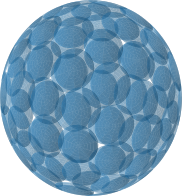Example:

import numpy as np

# scheme.show()
val = scheme.integrate(lambda x: np.exp(x), [0.0, 0.0, 0.0], 1.0)


Integration on the sphere can also be done for functions defined in spherical coordinates:

import numpy as np

def f(theta_phi):
theta, phi = theta_phi
return np.sin(phi) ** 2 * np.sin(theta)

val = scheme.integrate_spherical(f)


### Ball (S3)Example:

import numpy as np

# scheme.show()
val = scheme.integrate(lambda x: np.exp(x), [0.0, 0.0, 0.0], 1.0)


### Tetrahedron (T3)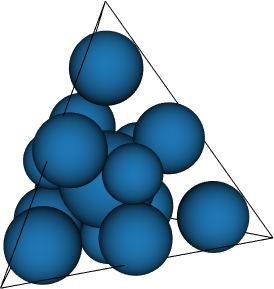Example:

import numpy as np

# scheme.show()
val = scheme.integrate(
lambda x: np.exp(x),
[[0.0, 0.0, 0.0], [1.0, 0.0, 0.0], [0.5, 0.7, 0.0], [0.3, 0.9, 1.0]],
)


### Hexahedron (C3)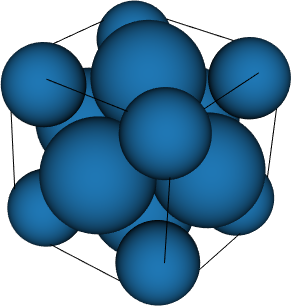Example:

import numpy as np

# scheme.show()
val = scheme.integrate(
lambda x: np.exp(x),
quadpy.c3.cube_points([0.0, 1.0], [-0.3, 0.4], [1.0, 2.1]),
)


### Pyramid (P3)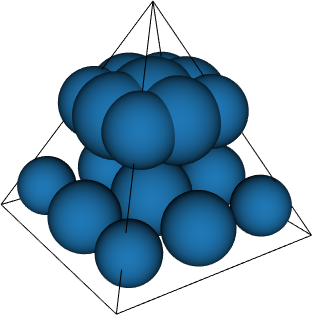• Felippa (2004, 9 schemes up to degree 5)

Example:

import numpy as np

val = scheme.integrate(
lambda x: np.exp(x),
[
[0.0, 0.0, 0.0],
[1.0, 0.0, 0.0],
[0.5, 0.7, 0.0],
[0.3, 0.9, 0.0],
[0.0, 0.1, 1.0],
],
)


### Wedge (W3)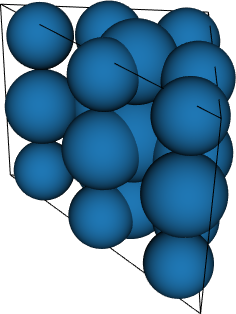Example:

import numpy as np

val = scheme.integrate(
lambda x: np.exp(x),
[
[[0.0, 0.0, 0.0], [1.0, 0.0, 0.0], [0.5, 0.7, 0.0]],
[[0.0, 0.0, 1.0], [1.0, 0.0, 1.0], [0.5, 0.7, 1.0]],
],
)


### 3D space with weight function exp(-r) (E3r)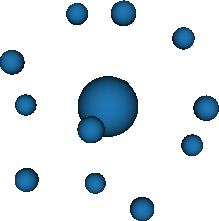Example:

import quadpy

# scheme.show()
val = scheme.integrate(lambda x: x ** 2)


### 3D space with weight function exp(-r2) (E3r2)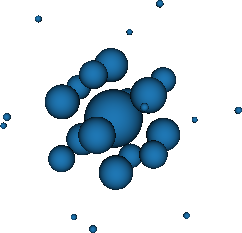Example:

import quadpy

# scheme.show()
val = scheme.integrate(lambda x: x ** 2)


### n-Simplex (Tn)

Example:

import numpy as np

dim = 4
val = scheme.integrate(
lambda x: np.exp(x),
np.array(
[
[0.0, 0.0, 0.0, 0.0],
[1.0, 2.0, 0.0, 0.0],
[0.0, 1.0, 0.0, 0.0],
[0.0, 3.0, 1.0, 0.0],
[0.0, 0.0, 4.0, 1.0],
]
),
)


### n-Sphere (Un)

Example:

import numpy as np

dim = 4
val = scheme.integrate(lambda x: np.exp(x), np.zeros(dim), 1.0)


### n-Ball (Sn)

Example:

import numpy as np

dim = 4
val = scheme.integrate(lambda x: np.exp(x), np.zeros(dim), 1.0)


### n-Cube (Cn)

Example:

import numpy as np

dim = 4
val = scheme.integrate(
lambda x: np.exp(x),
quadpy.cn.ncube_points([0.0, 1.0], [0.1, 0.9], [-1.0, 1.0], [-1.0, -0.5]),
)


### nD space with weight function exp(-r) (Enr)

Example:

import quadpy

dim = 4
val = scheme.integrate(lambda x: x ** 2)


### nD space with weight function exp(-r2) (Enr2)

Example:

import quadpy

dim = 4
val = scheme.integrate(lambda x: x ** 2)


## Project details

This version0.17.120.17.110.17.100.17.70.17.10.17.00.16.270.16.260.16.220.16.210.16.200.16.190.16.17

Uploaded py3